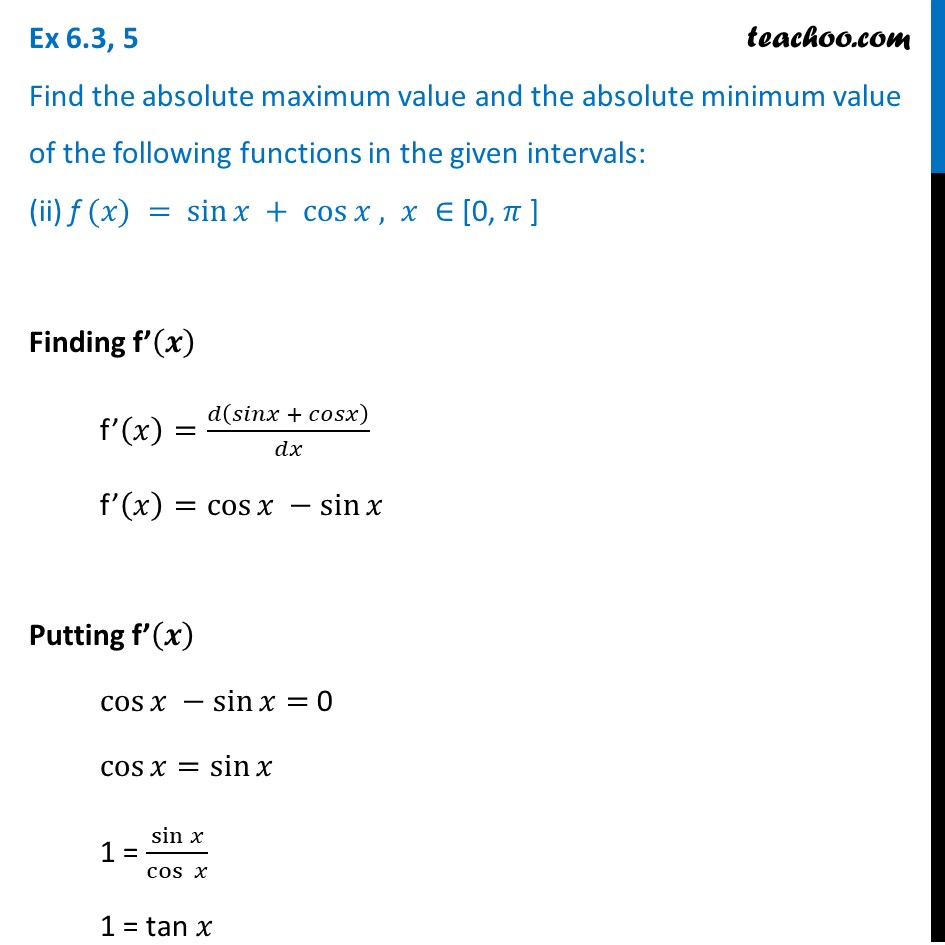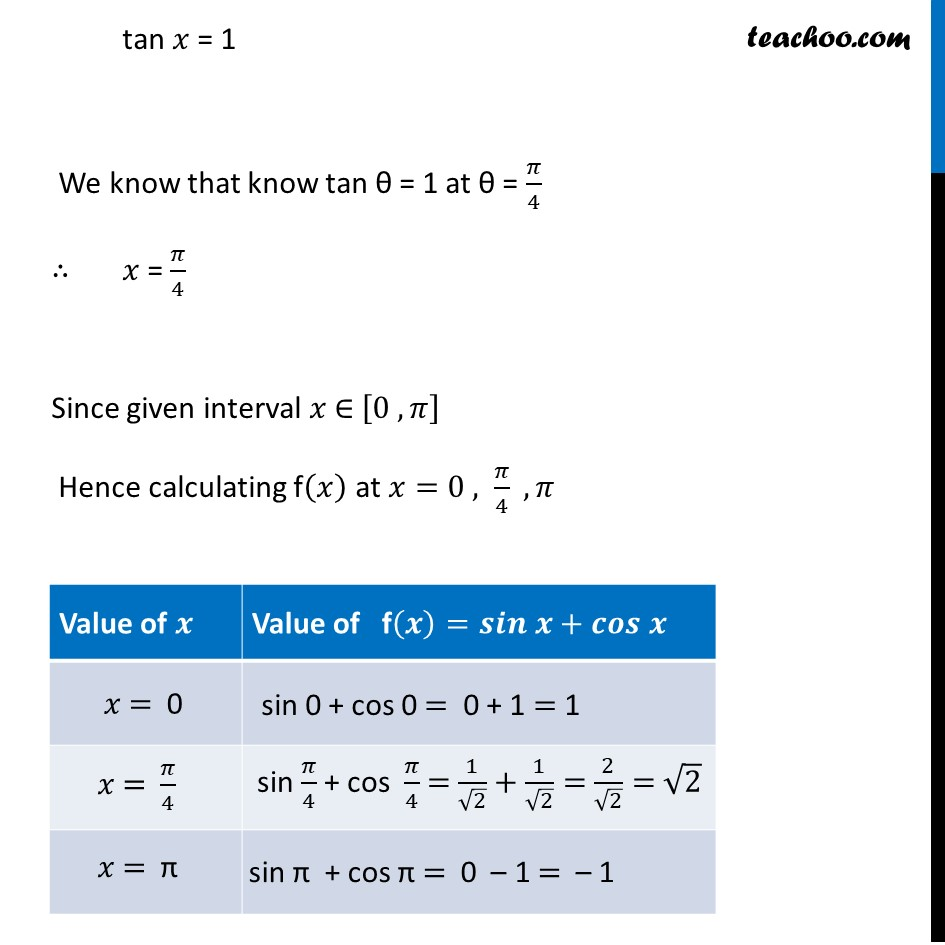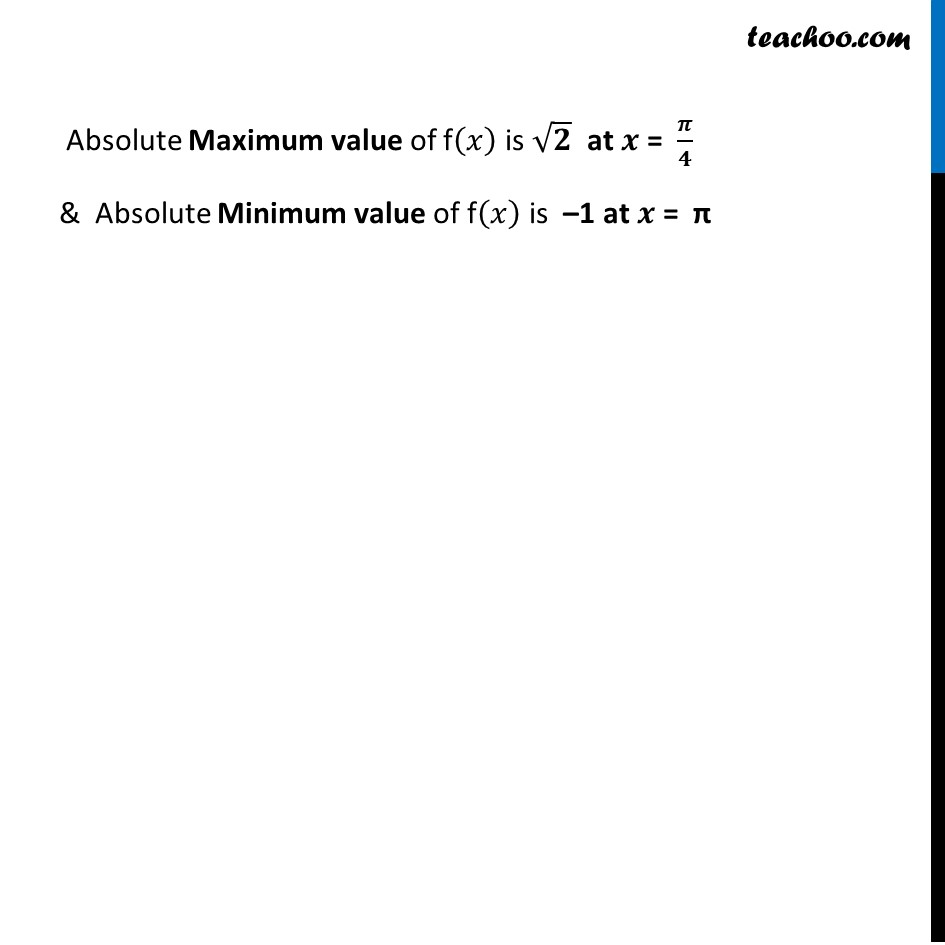Ex 6.3

Chapter 6 Class 12 Application of Derivatives
Serial order wiseLearn in your speed, with individual attention - Teachoo Maths 1-on-1 Class

### Transcript

Ex 6.3, 5 Find the absolute maximum value and the absolute minimum value of the following functions in the given intervals: (ii) f (𝑥) = sin⁡𝑥 + cos⁡𝑥 , 𝑥 ∈ [0, 𝜋 ] Finding f’(𝒙) f’(𝑥)=𝑑(𝑠𝑖𝑛𝑥 + 𝑐𝑜𝑠𝑥)/𝑑𝑥 f’(𝑥)=cos⁡〖𝑥 −sin⁡𝑥 〗 Putting f’(𝒙) cos⁡〖𝑥 −sin⁡𝑥 〗= 0 cos⁡〖𝑥=sin⁡𝑥 〗 1 = sin⁡𝑥/(cos⁡ 𝑥) 1 = tan 𝑥 tan 𝑥 = 1 We know that know tan θ = 1 at θ = 𝜋/4 ∴ 𝑥 = 𝜋/4 Since given interval 𝑥 ∈ [0 , 𝜋] Hence calculating f(𝑥) at 𝑥=0 , 𝜋/4 ,𝜋 Absolute Maximum value of f(𝑥) is √𝟐 at 𝒙 = 𝝅/𝟒 & Absolute Minimum value of f(𝑥) is –1 at 𝒙 = π